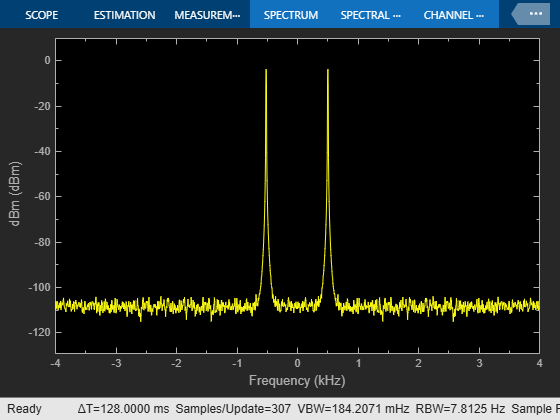Documentation

## Design an NCO Source Block

This example shows how to design an NCO source block with the following specifications:

• Desired output frequency:• Frequency resolution:• Spurious free dynamic range:• Sample period:• Desired phase offset:1. Calculate the number of required accumulator bits from the equation for frequency resolution:

``
``
``

Note that `N` must be an integer value. The value of `N` is rounded up to the nearest integer; 18 accumulator bits are needed to accommodate the value of the frequency resolution.

2. Using this best value of N, calculate the frequency resolution that will be achieved by the NCO block:

``
``
``

3. Calculate the number of quantized accumulator bits from the equation for spurious free dynamic range and the fact that for a lookup table with 2^P entries, P is the number of quantized accumulator bits:

``
``
``

4. Select the number of dither bits. In general, a good choice for the number of dither bits is the accumulator word length minus the number of quantized accumulator bits; in this case 4.

5. Calculate the phase increment:

phase increment =phase increment =phase increment = 16712

6. Calculate the phase offset,, using the desired phase offset,:

``
``
``

7. Open and simulate the model:8. Experiment with the model to observe the effects on the output shown on the Spectrum Analyzer. For example, try turning dithering on and off, and try changing the number of dither bits.